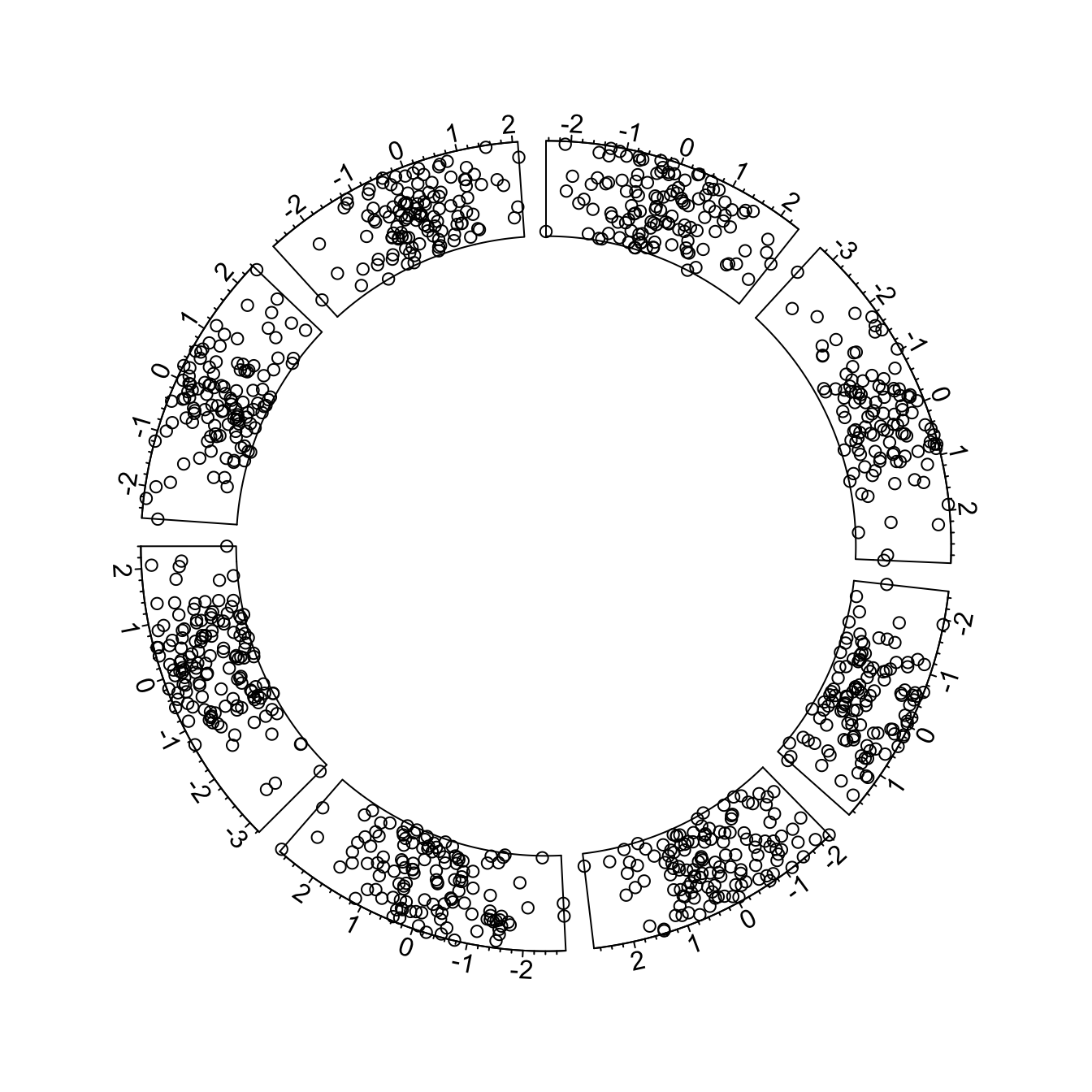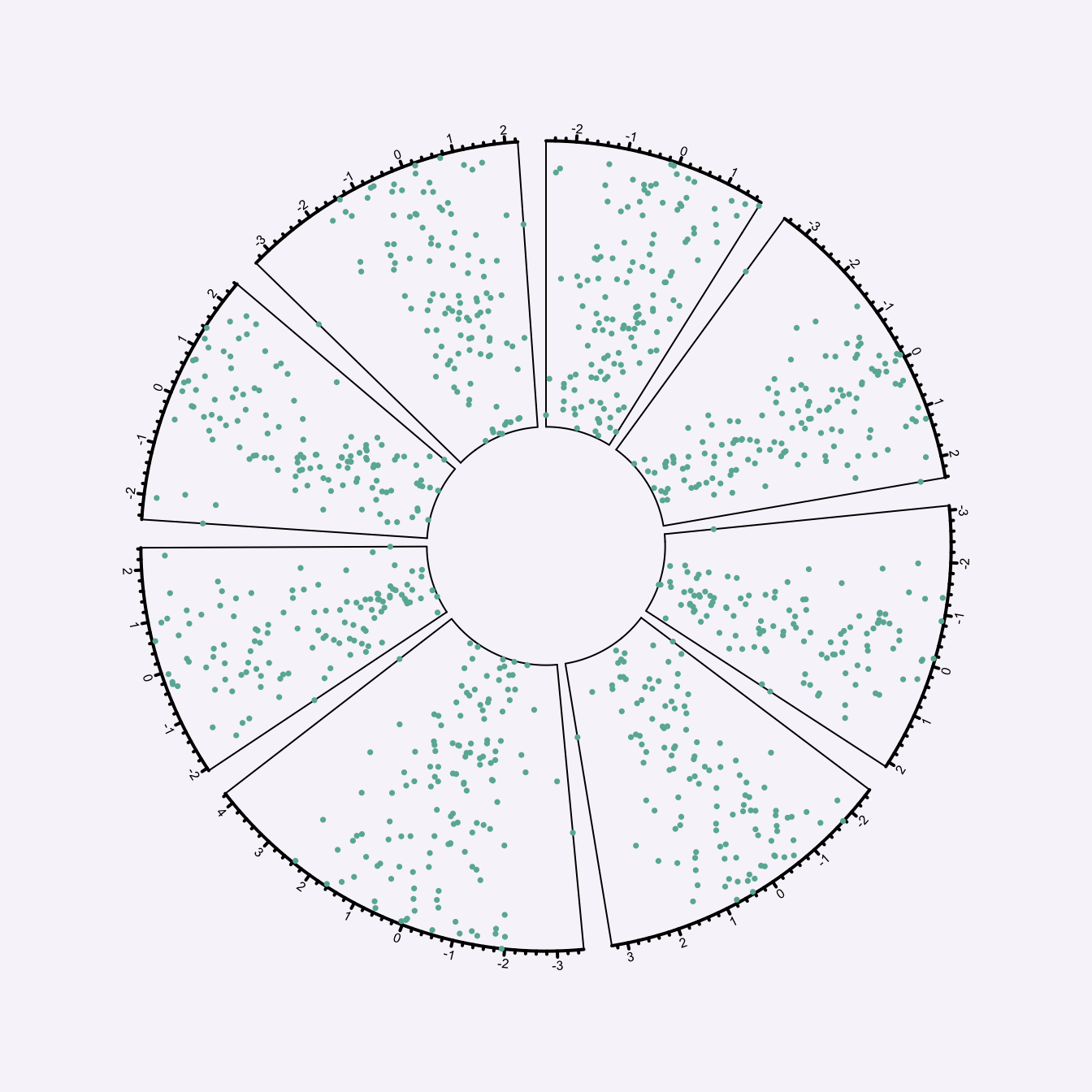# Circular chart customization for the circlize R package

This post follows the previous introduction to the `circlize` package. It shows how to apply basic customizations to a circular chart: how to change track width, background color, start angle and more.

# Most basic circular chart

Let’s remind how to build a basic circular chart with the `circlize` package.

This is extensively described in chart #224.``````# Upload library
library(circlize)

# Create data
data = data.frame(
factor = sample(letters[1:8], 1000, replace = TRUE),
x = rnorm(1000),
y = runif(1000)
)

# Step1: Initialise the chart giving factor and x-axis.
circos.initialize( factors=data\$factor, x=data\$x )

# Step 2: Build the regions.
circos.trackPlotRegion(factors = data\$factor, y = data\$y, panel.fun = function(x, y) {
circos.axis()
})

circos.trackPoints(data\$factor, data\$x, data\$y)``````

# Customization

Customization can happen at 3 different levels:

• initialization: use the usual `par()` and the specific `circos.par()` functions for general stuff.
• in `circos.axis()` to customize axis
• in circos.trackPoints()` to customize the chart shapes.

Note that most of the parameters are consistent with base R. Have a look to the scatterplot section of the gallery for more customization.``````# Upload library
library(circlize)

# Create data
data = data.frame(
factor = sample(letters[1:8], 1000, replace = TRUE),
x = rnorm(1000),
y = runif(1000)
)

# General Customization:
par(
mar = c(1, 1, 1, 1),           # Margin around chart
bg = rgb(0.4,0.1,0.7,0.05)     # background color
)
circos.par("track.height" = 0.6) # track hight, 0.6 = 60% of total height

# Step1: Initialise the chart giving factor and x-axis.
circos.initialize( factors=data\$factor, x=data\$x )

# Step2: Build regions.
circos.trackPlotRegion(factors = data\$factor, y = data\$y, panel.fun = function(x, y) {
circos.axis(
h="top",                   # x axis on the inner or outer part of the track?
labels=TRUE,               # show the labels of the axis?
major.tick=TRUE,           # show ticks?
labels.cex=0.5,            # labels size (higher=bigger)
labels.font=1,             # labels font (1, 2, 3 , 4)
direction="outside",       # ticks point to the outside or inside of the circle ?
minor.ticks=4,             # Number of minor (=small) ticks
major.tick.percentage=0.1, # The size of the ticks in percentage of the track height
lwd=2                      # thickness of ticks and x axis.
)
})